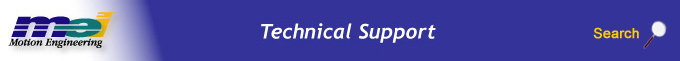.

Getting Started with MechaWare

Get Simulink to use MechaWare Blocks

1. Open Matlab. Change the current work directory to the Matlab\work\mechaware directory. In the example below, we selected C:\MATLAB6p5p1\work\mechaware by using the Current Directory drop down box.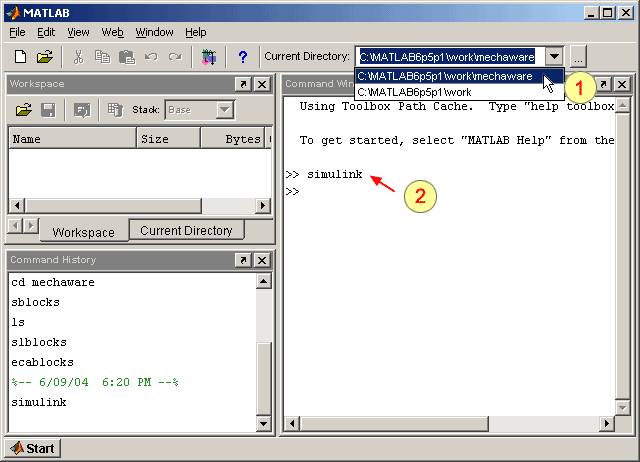2.  >>MechaWare Tip To keep from having to change the directory every time you use MechaWare, you can manually change the default path in Matlab. To learn how, click here.

3. In Simulink, click File > Open.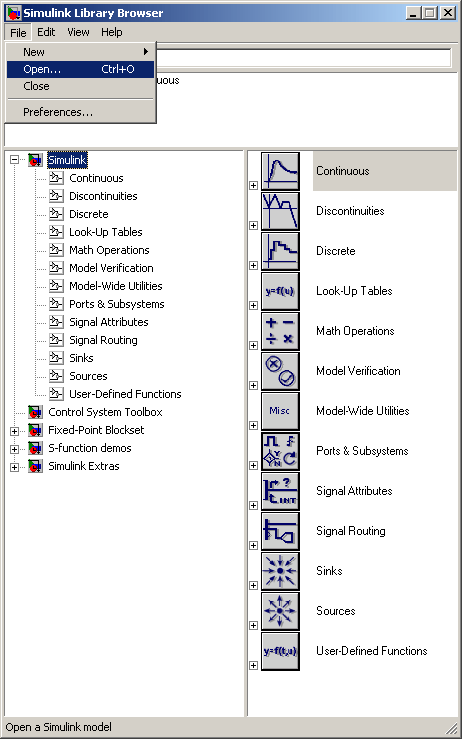4. Select ecalaw.mdl in your Matlab\work directory and click Open.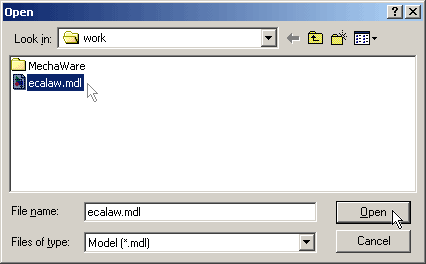5. You will now see ecalaw.mdl, which is a simple PID loop with a low pass 400 Hz corner frequency.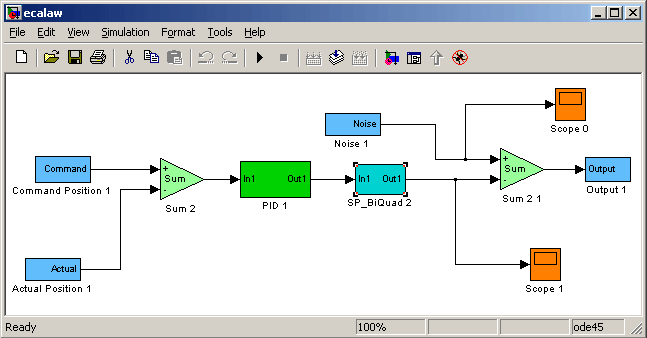Using MechaWare Blocks

Now that we have the sample control loop ecalaw.mdl open, let's look into how the control loop is made up of different basic blocks. The screenshot of ecalaw.mdl below should look familiar since it's a slightly modified PID loop.Here is a quick breakdown of the blocks in the diagram above.

NOTE: The titles below the blocks are generic names and can be changed by the user to any Simulink-valid name.

Command Position 1
The Command Position actually represents the command position of the specified Axis. In our example, Command Position 1 represents the command position of Axis 0.

If you double-click on the Command Position 1 block you will notice that the Command Position is coming from Axis 0. In this example, the Axis Number is set to COMMAND0. However, if we wanted the Command Position from Axis 4, we can change the Axis Number and set it to COMMAND4.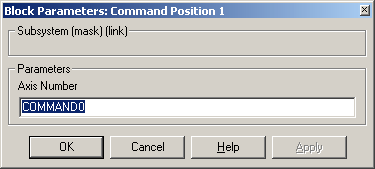Actual Position 1

Similar to Command Position, Actual Position actually represents the Actual Position of the specified Axis.

If you double-click on the Actual Position 1 block you will notice that the Actual Position is coming from Axis 0. In this example, the Axis Number is set to ACTUAL0. However, if we wanted the Actual Position from Axis 4, we can change the Axis Number and set it to ACTUAL4.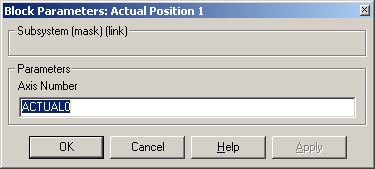Sum2

This is a summing block with variable gains on each input. Double-click on the Sum 2 block to adjust the gains.
K1 sets the top input gain (+ label on the block).
K2 sets the bottom gain (-1 label on the block).
K1 = 1 and K2 = -1, makes the summing block a difference block.PID 1

Double-clicking on the PID 1 block will show the PID gains in the PID block. The PID gains are consistent with the standard PID loop in the XMP. The variable Ts is the sample time of the system and is only used in Simulink simulation—it is never used on the controller.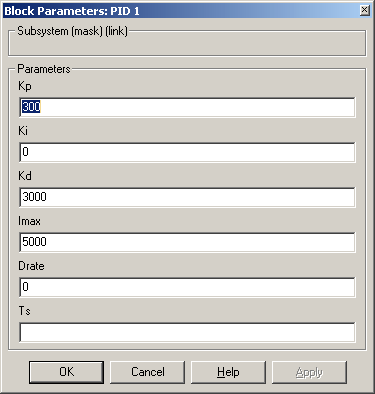This block is a biquad (2nd order digital filter). Double-clicking on this block shows two variables: Biquad Structure and Ts.

The Biquad Structure is the structure that specifies the filter to be used. In this case it looks for lp400.txt. We will discuss how to set these filters in a later section.

Ts is the sample time of the system in seconds. Ts is only used in Simulink simulation—it is never loaded to the controller.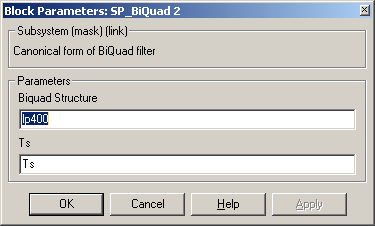Output 1
The Output 1 block writes the result to the torque command (i.e. DAC Output) to the motor specified. Double-click on the Output 1 block to set the motor that is used in this block. In our example, MOTOR0 sends torque commands to Motor 0. Setting it to MOTOR5 would send torque commands to Motor 5.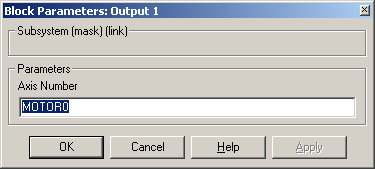Legal Notice  |  Tech Email  |  Feedback        Copyright © 2001-2010 Motion Engineering